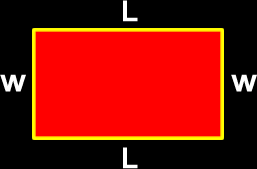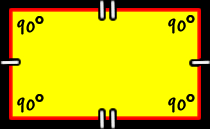# The Properties of a Rectangle

Definitions and formulas for the perimeter of a rectangle, the area of a rectangle,  how to find the length of the diagonal of a rectangle, properties of the diagonals of a rectangle

Just scroll down or click on what you want and I'll scroll down for you!

 perimeter of a rectangle area of a rectangle properties of the sidesand angles of a rectangle diagonal of a rectangle properties of the diagonalsof a rectangle

 The perimeter of a rectangle:To find the perimeter of a rectangle, just add up all the lengths of the sides:Perimeter = L + w + L + w        = 2L + 2wThe area of a rectangle:To find the area of a rectangle, just multiply the length times the width:Area = L x wThe sides and angles of a rectangle:Opposite sides of a rectangle are the same length (congruent). The angles of a rectangle are all congruent (the same size and measure.) Remember that a 90 degree angle is  called a "right angle."  So, a rectangle has four right angles. Opposite angles of a rectangle are congruent.Opposite sides of a rectangle are parallel.